Function Repository Resource:

# MultiplicativeDigitalRoot

Compute the multiplicative digital root, in any base, of an integer

Contributed by: Christopher Stover
 ResourceFunction["MultiplicativeDigitalRoot"][n,b] gives the multiplicative digital root of the non-negative integer n when expressed in the base b. ResourceFunction["MultiplicativeDigitalRoot"][n] gives the base-10 muliplicative digital root of n.

## Details

Starting with a non-negative integer n, multiply its base-b digits, then multiply the digits of the resulting number, etc., until the result has only one digit. The one-digit integer marking the stopping point of this process is called the multiplicative digital root of n.
As an example in base 10, consider n=14691:
Multiplying its digits yields 1×4×6×9×1=216.
Multiplying the digits of the result yields 2×1×6=12.
Multiplying the digits of the result yields 1×2=2. Because 2 is a single-digit number, the process stops.
Because the integer marking the termination of the process is 2, ResourceFunction["MultiplicativeDigitalRoot"] returns 2.

## Examples

### Basic Examples (2)

Compute the multiplicative digital root of 1191:

 In:=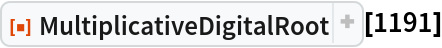Out=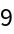Compute the additive digital root of 1191, base-14:

 In:=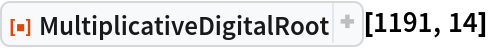Out=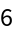### Scope (2)

 In:=Out=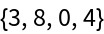In:=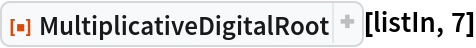Out=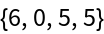Compute the multiplicative digital root of the first 100 integers (OEIS A031347):

 In:=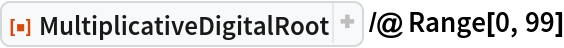Out=### Properties and Relations (3)

The result returned by MultiplicativeDigitalRoot can be iteratively computed using NestWhileList:

 In:=Out=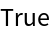The number of iterations required to reach the end of the digit multiplication process is called the multiplicative persistence and is returned by the resource function MultiplicativePersistence:

 In:=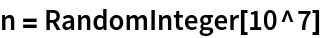Out=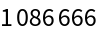In:=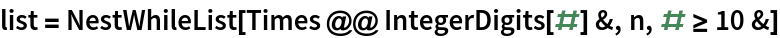Out=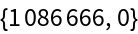In:=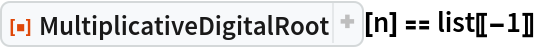Out=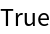In:=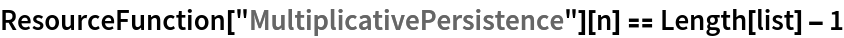Out=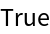The additive analogue of the multiplicative digital root is called the additive digital root and is returned by the resource function AdditiveDigitalRoot:

 In:=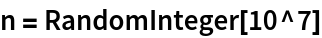Out=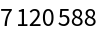In:=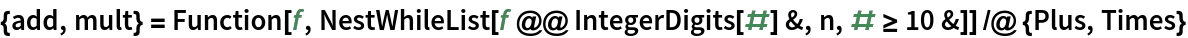Out=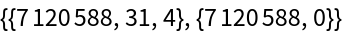In:=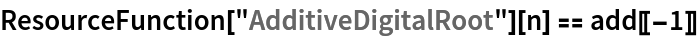Out=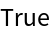### Possible Issues (1)

MultiplicativeDigitalRoot requires its input to be non-negative:

 In:=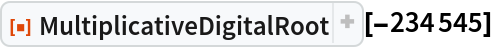Out=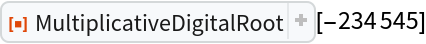### Neat Examples (1)

Create a OEIS-themed table showing which integers have the same multiplicative digital root. This partially reproduces a cool result from the associated MathWorld article:

 In:=Out=## Version History

• 1.1.0 – 25 May 2023
• 1.0.0 – 09 August 2022

## Author Notes

As mentioned on the page Problems & Puzzles, Puzzle 341, there is a modified definition of multiplicative persistence due to Erdős wherein one only multiplies the nonzero digits at each step. This modification is not implemented herein but may be included in a future update.
In a future update, I'm going to make the "Neat Examples" table look better. That's definitely a version 2 edit!